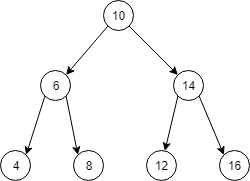# 二叉搜索树的后序遍历序列

### 代码

public class Solution {
public boolean VerifySquenceOfBST(int [] sequence) {
if(sequence == null || sequence.length == 0) return false;
return func(sequence, 0, sequence.length - 1);
}

private boolean func(int[] array, int l, int r){
if(l == r){
return true;
}
int i = l;
while(array[i] < array[r]){
i++;
}
int k = i;
while(array[k] > array[r]){
k++;
}
boolean left = true;
boolean right = true;
if(i > l){
left = func(array, l, i - 1);
}
if(k > i){
right = ((k == r) && func(array, i, r - 1));
}
//防止第一个array[l]过大, 原地踏步. 不加也可以跑过牛客网的用例
if(i == k && i != r){
return false;
}
return left && right;
}
}


### 思路if(i == k && i != r){
return false;
}